﻿ Year 2 Addition and Subtraction (5)
 HOME ABOUT US SHOP PRIVACY CONTACT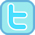Year 1 Year 2 Year 3 Year 4 Year 5 Year 6

# Year 2 Addition and Subtraction (5)

### Pupils should be taught to recognise and use the inverse relationship between addition and subtraction and use this to check calculations and solve missing number problems.

 Our printable resources...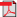Inverting Addition Mad Maths Minutes (grey) Sets A & B          Sets C & DInverting Addition Mad Maths Minutes (black) Sets A & B          Sets C & DInverting Subtraction Mad Maths Minutes (grey) Sets A & B          Sets C & DInverting Subtraction Mad Maths Minutes (black) Sets A & B          Sets C & D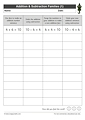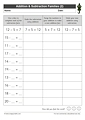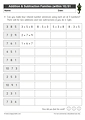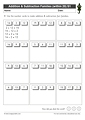Addition & Subtraction Fact Families (1)Addition & Subtraction Fact Families (2)Addition & Subtraction Fact Families (within 10) Sheet 1Addition & Subtraction Fact Families (within 10) Sheet 2Addition & Subtraction Fact Families (within 20) Sheet 1Addition & Subtraction Fact Families (within 20) Sheet 2Addition & Subtraction Fact Families (3)Addition & Subtraction Inverses (Inverting Addition)Addition & Subtraction Inverses (Inverting Subtraction)Our online activities...Addition & Subtraction Inverses to 10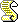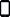Addition and Subtraction Fact FamiliesI think of a number (addition/subtraction within 20)Addition and Subtraction Fact Families (numbers to 99)Links to sites that we like...EZ School Addition & Subtraction Fact Family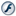TES iBoard - Inverse Relationships (Addition & Subtraction)